# Introduction

This document provides programmatic solutions in the R package for statistical computing for many of the exercises in “Causal Inference in Statistics: A Primer” by Pearl, Glymour, and Jewell.

To get the most out of the exercises, by all means solve them first using pen and paper. Once you’ve accomplished that, use this document to:

• Learn how you can solve these tasks programmatically in the R environment.

Without doubt, after learning about causal inference from the book, you will be keen to apply these methods to your own research questions. Sometimes you will then be facing much larger graphs than those used in the book (like, for instance, the examples posted on http://www.dagitty.net). The computational recipes shown in this document all scale up to large graphs. In the long term you will find convenient, and less error-prone, to solve causal inference problems with the help of software.

What you need to follow these instructions is a working installation of R with the following two packages installed:

• dagitty - a package for structural causal models.
• lavaan - a package for structural equation modeling.
library(dagitty)
library(lavaan)

# Preliminaries

## Study question 1.4.1

We first define the graph in this exercise using dagitty syntax and plot it.

g <- dagitty('dag {
X [pos="0,1"]
Y [pos="1,1"]
Z [pos="2,1"]
W [pos="1,0"]
T [pos="2,2"]

X -> Y -> Z -> T
X -> W -> Y -> T
W -> Z
}')
plot(g)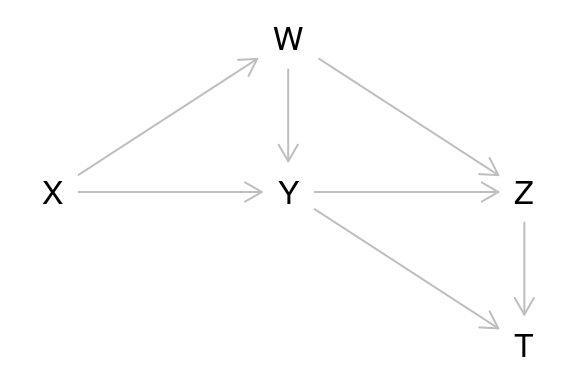This question introduces us to the “ancestry”-related terminology that is commonly applied to graphical models. For each of the relationships discussed, dagitty has a corresponding command, which makes solving (a) to (d) straightforward:

1. Name all of the parents of $$Z$$.
parents( g, "Z" )
##  "W" "Y"
1. Name all of the ancestors of $$Z$$.
ancestors( g, "Z" )
##  "Z" "Y" "X" "W"
1. Name all of the children of $$W$$.
children( g, "W" )
##  "Y" "Z"
1. Name all of the descendants of $$W$$.
descendants( g, "W" )
##  "W" "Z" "T" "Y"
1. Draw all (simple) paths between $$X$$ and $$T$$.

For detailed inspection of paths and their statuses, dagitty provides the function paths. This function returns a list with two components, paths and open; the second component is not relevant for this exercise. So we invoke paths in the following manner:

paths( g, "X", "T" )$paths ##  "X -> W -> Y -> T" "X -> W -> Y -> Z -> T" "X -> W -> Z -> T" ##  "X -> W -> Z <- Y -> T" "X -> Y -> T" "X -> Y -> Z -> T" ##  "X -> Y <- W -> Z -> T" 1. Draw all the directed paths between $$X$$ and $$T$$. This is achieved simply by passing the option directed=TRUE to the paths command. paths( g, "X", "T", directed=TRUE )$paths
##  "X -> W -> Y -> T"      "X -> W -> Y -> Z -> T" "X -> W -> Z -> T"
##  "X -> Y -> T"           "X -> Y -> Z -> T"

## Study question 1.5.1

This question is are analytical in nature. What we shall do here is verify our answers by simulation. First, let us generate some data by directly implementing the model specification.

N <- 10000 # sample size
Ux <- rnorm( N ); Uy <- rnorm( N ); Uz <- rnorm( N )
X <- Ux
Y <- 1/3*X + Uy
Z <- 1/16*Y + Uz
d <- data.frame(X=X,Y=Y,Z=Z)
1. Draw the graph that complies with the model.

We use this exercise to introduce another option to plot graphs in dagitty: specify the graph itself and the graph layout in two separate commands.

g <- dagitty("dag {
Ux -> X -> Y -> Z <- Uz
Uy -> Y
}")
coordinates(g) <- list(
x=c(Ux=1,Uy=2,Uz=3,X=1,Y=2,Z=3),
y=c(Ux=1,Uy=1,Uz=1,X=0,Y=0,Z=0) )
plot(g)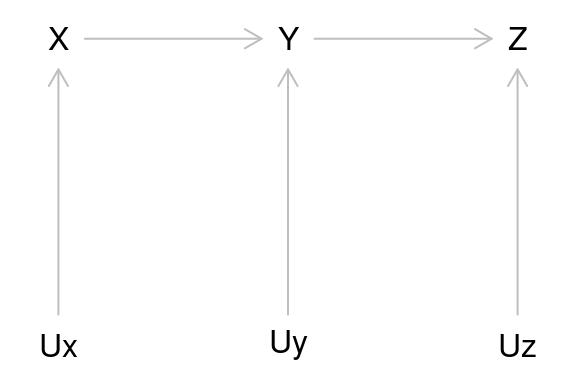1. Determine the best guess of the value (expected value) of $$Z$$, given that we observe $$Y=3$$.

The answer should be 3/16. So the following expression should return a value close to 3:

16 * predict(lm(Z~Y,d),list(Y=3),interval="confidence")
##        fit      lwr     upr
## 1 2.659431 1.716622 3.60224
1. Determine the best guess of the value of Z, given that we observe X=3.

This should be 1/16, so the following should give us a value close to 1:

16 * predict(lm(Z~X,d),list(X=3),interval="confidence")
##        fit      lwr      upr
## 1 1.233844 0.216446 2.251242
1. Determine the best guess of the value of $$Z$$, given that we observe $$X=1$$ and $$Y=3$$.

If we observe that $$Y=3$$, then the fact that $$X=1$$ becomes irrelevant for predicting $$Z$$. So the answer should be 3, just like in (b).

16 * predict(lm(Z~X+Y,d),list(X=1,Y=3),interval="confidence")
##        fit      lwr      upr
## 1 2.674647 1.730899 3.618395
1. Determine the best guess of $$X$$, given that we observed $$Y=2$$.
cov(d)
##           X          Y          Z
## X 0.9655283 0.33092299 0.02534720
## Y 0.3309230 1.13530374 0.06376759
## Z 0.0253472 0.06376759 1.02474975

The regression coefficient of $$X \sim Y$$ should be $$\frac{1}{3} / (1+\frac{1}{9}) = \frac{9}{30} = 0.3$$. So the best guess should be $$0.6$$.

predict(lm(X~Y,d),list(Y=2),interval="confidence")
##         fit       lwr       upr
## 1 0.5862489 0.5476096 0.6248882

Determine the best guess of $$Y$$, given that we observed $$X=1$$ and $$Z=3$$.

Finding this value requires an involved calculation, which gives an answer close to (but not equal to) 0.5. In case you wish to verify your result, here’s how:

predict(lm(Y~X+Z,d),list(X=1,Z=3),interval="confidence")
##         fit       lwr       upr
## 1 0.5152635 0.4506377 0.5798892

# Graphical Models and Their Applications

## Study question 2.3.1

First we define the two graphs used in this exercise.

fig2.5 <- dagitty("dag {
X -> R -> S -> T <- U <- V -> Y
}")
plot( graphLayout( fig2.5 ) )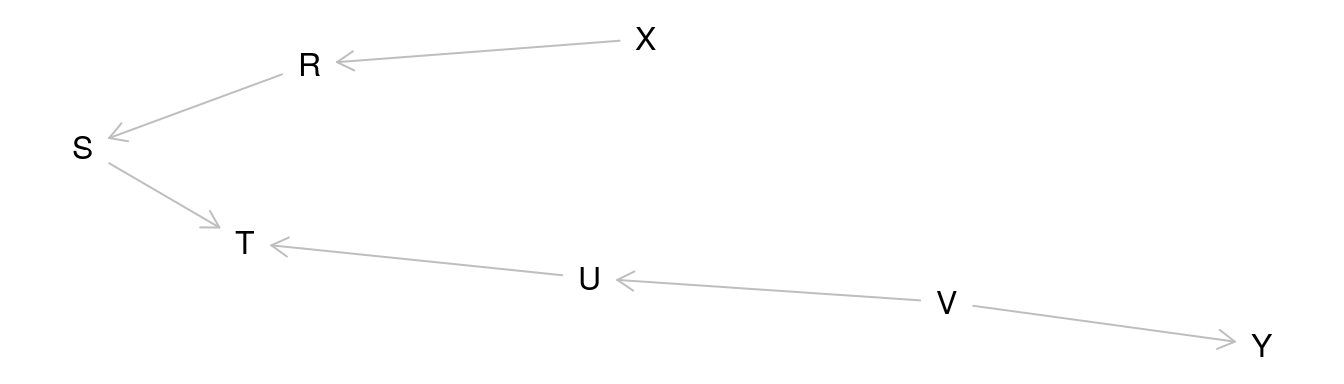fig2.6 <- dagitty("dag {
X -> R -> S -> T <- U <- V -> Y
T -> P
}")
plot( graphLayout( fig2.6 ) )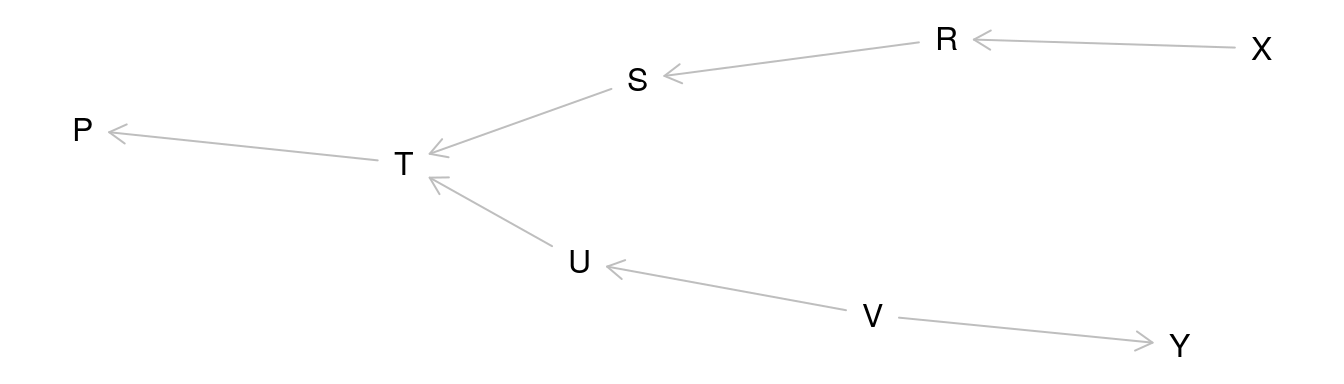1. List all pairs of variables in the graph that are independent conditional on the set $$Z=\{R, V\}$$.

We can determine whether two variables $$X$$ and $$Y$$ are independent given $$\{R, V\}$$ by inspecting the path between $$X$$ and $$Y$$. For example, $$X$$ and $$Y$$ are independent because the path between them is closed given $$\{R, V\}$$:

paths( fig2.5, "X", "Y", c("R","V") )
## $paths ##  "X -> R -> S -> T <- U <- V -> Y" ## ##$open
##  FALSE

To answer the question programmatically, we just do this for all possible pairs of variables (except $$R$$ and $$V$$):

pairs <- combn( c("X","S","T","U","Y"), 2 )
apply( pairs, 2, function(x){
p <- paths( fig2.5, x, x, c("R","V") )
if( !p$open ){ message( x," and ",x," are independent given {R,V}" ) } else { message( x," and ",x," are possibly dependent given {R,V}" ) } } ) ## X and S are independent given {R,V} ## X and T are independent given {R,V} ## X and U are independent given {R,V} ## X and Y are independent given {R,V} ## S and T are possibly dependent given {R,V} ## S and U are independent given {R,V} ## S and Y are independent given {R,V} ## T and U are possibly dependent given {R,V} ## T and Y are independent given {R,V} ## U and Y are independent given {R,V} Note that this code relies on the fact that there’s only one path between each pair of variables. Otherwise, the check if( !p$open )

would not be sufficient.

1. For each pair of non-adjacent variables, give a set of variables that, when conditioned on, renders that pair independent.

We could solve this by checking for each possible set whether that set renders the pair of variables independent. But this would be quite cumbersome already for this small graph. Alternatively, we can use a dagitty function.

impliedConditionalIndependencies( fig2.5 )
##   "R _||_ T | S" "R _||_ U"     "R _||_ V"     "R _||_ Y"
##   "S _||_ U"     "S _||_ V"     "S _||_ X | R" "S _||_ Y"
##   "T _||_ V | U" "T _||_ X | R" "T _||_ X | S" "T _||_ Y | V"
##  "T _||_ Y | U" "U _||_ X"     "U _||_ Y | V" "V _||_ X"
##  "X _||_ Y"

This function lists, for each non-adjacent pair of variables, all minimal sets that we can condition on to render that pair independent. In this example, we never need to condition on more than one variable. But as we know from the previous exercise, larger conditioning sets (two variables and more) can also be necessary. Dagitty does not list those because the result would quickly grow very large for graphs with more variables.

1. List all pairs of variables in the graph of Figure 2.6 that are independent conditional on the set $$Z = \{R, P\}$$.

We could do this in the same way as in (a). However, now we are going to use a different method that does not rely on listing and inspecting paths; an advantage of that method is that it scales up to larger graphs, in which we might have to check millions of paths. Our solution is based on the function dseparated, which tests whether there is any open path between two variables of interest rather than listing them all. To keep the output short, we list only the independent variable pairs.

pairs <- combn( c("X","S","T","U","Y","V"), 2 )
apply( pairs, 2, function(x){
if( dseparated( fig2.6, x, x, c("R","P") ) ){
message( x," and ",x," are independent given {R,P}" )
}
} )
## X and S are independent given {R,P}
## X and T are independent given {R,P}
## X and U are independent given {R,P}
## X and Y are independent given {R,P}
## X and V are independent given {R,P}

In particular, $$S$$ and $$U$$ are now dependent because we condition on $$P$$; they were still independent in (a).

1. For each pair of non-adjacent variables in in Figure 2.6, give a set of variables that, when conditioned on,renders that pair independent.

This one is solved in exactly the same way as (b):

impliedConditionalIndependencies( fig2.6 )
##   "P _||_ R | S" "P _||_ R | T" "P _||_ S | T" "P _||_ U | T"
##   "P _||_ V | U" "P _||_ V | T" "P _||_ X | R" "P _||_ X | S"
##   "P _||_ X | T" "P _||_ Y | V" "P _||_ Y | U" "P _||_ Y | T"
##  "R _||_ T | S" "R _||_ U"     "R _||_ V"     "R _||_ Y"
##  "S _||_ U"     "S _||_ V"     "S _||_ X | R" "S _||_ Y"
##  "T _||_ V | U" "T _||_ X | R" "T _||_ X | S" "T _||_ Y | V"
##  "T _||_ Y | U" "U _||_ X"     "U _||_ Y | V" "V _||_ X"
##  "X _||_ Y"
1. Suppose we generate data by the model described in Figure 2.5, and we fit them with the linear equation $$Y = a + bX + cZ$$. Which of the variables in the model may be chosen for $$Z$$ so as to guarantee that the slope b would be equal to zero?

We can translate this question into: For which variables $$Z$$ are $$X$$ and $$Y$$ d-separated given $$Z$$? We can find these out by simply testing d-separation for all variables in the graph:

sapply( names(fig2.5), function(Z) dseparated(fig2.5,"X","Y",Z) )
##     R     S     T     U     V     X     Y
##  TRUE  TRUE FALSE  TRUE  TRUE  TRUE  TRUE

As we can see, $$X$$ and $$Y$$ are independent given all variables except $$T$$. We can validate this result by generating data from the graph.

d <- simulateSEM( fig2.5, .7, .7, N=10000 )

The simulateSEM function chooses path coefficients for the model uniformly from the interval given by the second and third parameters. The given values sets this interval ta the single value 0.7, which ensures that the correlations in the generated data are strong enough to be picked up. Now, we validate our solution:

confint( lm( Y~X+V, data=d ) )
##                   2.5 %       97.5 %
## (Intercept) -0.02069436 0.0071808177
## X           -0.02701217 0.0007791438
## V            0.69215605 0.7201024493

Indeed, $$Y$$ and $$X$$ remain independent conditional on $$V$$.

confint( lm( Y~X+T, data=d ) )
##                   2.5 %       97.5 %
## (Intercept) -0.02830774  0.008273954
## X           -0.16217732 -0.123359230
## T            0.36380455  0.402466219

Conditioning on $$T$$ generates a negative association between $$Y$$ and $$X$$.

1. Continuing question (e), suppose we fit the data with the equation: $Y = a + bX + cR + dS + eT + fP$ which of the coefficients would be zero?

This question can be translated to: which of the five variables $$X, R, S, T, P$$ are d-separated from $$Y$$ given the same five variables? We can answer this question by invoking the function dseparated in a different way, namely by supplying the empty set as argument $$Y$$. This way, instead of checking whether given variables $$X, Y$$ are d-separated by $$Z$$, it returns the set of variables that are d-separated from $$X$$ by $$Z$$.

predictors <- c("X","R","S","T","P")
intersect( predictors, dseparated( fig2.6, "Y", list(), predictors ) )
##  "X" "R" "P"

Thus, it would seem that the slopes of $$X$$, $$R$$ and $$P$$ in this regression should be 0. This, too, can be verified in simulated data:

d <- simulateSEM( fig2.6, .7, .7, N=10000 )
confint( lm( Y ~ X + R + S + T + P, data=d ) )
##                     2.5 %      97.5 %
## (Intercept) -0.0200522452  0.01434711
## X           -0.0315338878  0.01645683
## R            0.0005617843  0.05998850
## S           -0.5362254072 -0.47724979
## T            0.6463064594  0.70455153
## P           -0.0157103883  0.03296456

Indeed, the confidence intervals for $$X$$, $$R$$ and $$P$$ all include 0 whereas those for $$S$$ and $$T$$ does not.

## Study question 2.4.1

First we define the graph of this question:

fig2.9 <- dagitty("dag {
X <- Z1 -> Z3 <- Z2 -> Y
X <- Z3 -> Y
X -> W -> Y
}")
coordinates(fig2.9) <- list(x=c(X=1,W=2,Y=3,Z1=1,Z3=2,Z2=3),
y=c(X=0,W=0,Y=0,Z2=-2,Z1=-2,Z3=-1))
plot(fig2.9)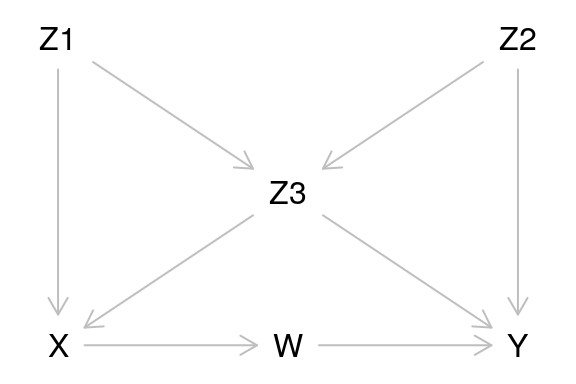1. For each pair of non-adjacent nodes in this graph, find a set of variables that d-separates that pair. What does this list tell us about independencies in the data?
impliedConditionalIndependencies( fig2.9 )
## W _||_ Z1 | X
## W _||_ Z2 | Z1, Z3
## W _||_ Z2 | X
## W _||_ Z3 | X
## X _||_ Y | W, Z2, Z3
## X _||_ Y | W, Z1, Z3
## X _||_ Z2 | Z1, Z3
## Y _||_ Z1 | X, Z2, Z3
## Y _||_ Z1 | W, Z2, Z3
## Z1 _||_ Z2
1. Repeat question (a) assuming that only variables in the set $$\{Z_3, W, X, Z_1\}$$ can be measured.
latents( fig2.9 ) <- setdiff( names(fig2.9), c("Z3", "W", "X", "Z1") )
impliedConditionalIndependencies( fig2.9 )
## W _||_ Z1 | X
## W _||_ Z3 | X
1. For each pair of non-adjacent nodes in the graph, determine whether they are independent conditional on all other variables.
pairs <- combn( names( fig2.9 ), 2 )
apply( pairs, 2, function(x){
all.other.variables <- setdiff( names(fig2.9), x )
if( dseparated( fig2.9, x, x, all.other.variables ) ){
message( x," and ",x," are independent given ",
paste( all.other.variables, collapse=",") )
}
} )
## W and Z1 are independent given X,Y,Z2,Z3
## X and Y are independent given W,Z1,Z2,Z3
## X and Z2 are independent given W,Y,Z1,Z3
## Y and Z1 are independent given W,X,Z2,Z3
1. For every variable $$V$$ in the graph, find a minimal set of nodes that renders $$V$$ independent of all other variables in the graph.

The set of all parents, all children, and all children’s parents of a variable is guaranteed to separate $$V$$ from all other variables. It is also guaranteed to be minimal: Parents and children must be included, and including the children means that their parents must also be included.

In the literature, that set is known as a variable’s Markov blanket.

for( v in names( fig2.9 ) ){
cat( "Variable", v, ":", markovBlanket( fig2.9, v ), "\n" )
}
## Variable W : X Y Z2 Z3
## Variable X : Z1 Z3 W
## Variable Y : W Z2 Z3
## Variable Z1 : X Z3 Z2
## Variable Z2 : Y Z3 W Z1
## Variable Z3 : Z1 Z2 X Y W
1. Suppose we wish to estimate the value of $$Y$$ from measurements taken on all other variables in the model. Find the smallest set of variables that would yield as good an estimate as before.
predictors <- setdiff( names( fig2.9 ), "Y" )
intersect( predictors, dconnected( fig2.9, "Y", list(), predictors ) )
##  "W"  "Z2" "Z3"

In general, this set would be the Markov blanket of $$Y$$. In this specific case, the Markov blanket is just the set of the parents of $$Y$$.

1. Repeat Question (e) assuming that we wish to estimate the value of $$Z_2$$.
predictors <- setdiff( names( fig2.9 ), "Z2" )
intersect( predictors, dconnected( fig2.9, "Z2", list(), predictors ) )
##  "W"  "Y"  "Z1" "Z3"

Again, the genereal answer is the Markov blanked of $$Z_2$$. In this case, the Markov blanket consists of the children of $$Z_2$$ together with their parents (except $$Z_2$$ itself). Note that conditioning on only the children would not suffice.

1. Suppose we wish to predict the value of $$Z_2$$ from measurements of $$Z_3$$. Would the quality of our prediction improve if we add measurement of $$W$$? Explain.

This is a tough question. Statistically speaking, it is well known that a (within-sample) prediction can never get worse by adding more predictors. However, we have learnt before that it does not help to add predictors that are d-separated from the outcome variable by the other predictors (in other words, once we include all variables in the Markov blanket prediction, we are good). So the first thing to check is whether $$W$$ is d-separated from $$Z_2$$ given $$Z_3$$. This is not the case, as can be easily seen by path inspection:

p <- paths( fig2.9, "Z2", "W", "Z3" )
p$paths[p$open]
##  "Z2 -> Z3 <- Z1 -> X -> W"

So, indeed, we expect to explain more variance in $$Z_2$$ by regressing it on both $$Z_3$$ and $$W$$ than by regressing on $$Z_3$$ alone. Let us verify this by simulation:

d <- simulateSEM( fig2.9, .4, .4, N=10000 )
summary( lm( Z2 ~ Z3, d ) )$r.squared ##  0.1623383 summary( lm( Z2 ~ Z3 + W, d ) )$r.squared
##  0.1625359

We can indeed see that the explained variance increases when including $$W$$, and the coefficient of $$W$$ is significant. However, the effect is tiny because the coefficient of $$W$$ in the regression model is much smaller than that of $$Z_3$$:

coef( lm( Z2 ~ Z3 + W, d ) )
## (Intercept)          Z3           W
## -0.01043136  0.40554947 -0.01464866

If this does not leave you convinced, it may be useful to illustrate the same principle in a simple collider model like so:

d <- simulateSEM( "dag{ x -> m <- y}", .7, .7 )
summary( lm( y ~ m, d ) )$r.squared ##  0.4858533 summary( lm( y ~ m + x, d ) )$r.squared
##  0.9610011

In this smaller example, the correlations are stronger, and so it more clearly illustrates the principle that adding an uncorrelated variable can nevertheless improve prediction of $$x$$ (observe that R-squared almost doubles).

## Study question 2.5.1

1. Which of the arrows in Figure 2.9 can be reversed without being detected by any statistical test? [Hint: Use the criterion for equivalence class.]

There are two ways to solve this question. One is simply to enumerate the equivalence class and see how many items it contains.

length(equivalentDAGs( fig2.9 ))
##  1

It seems that we have only one equivalent DAG! To verify this, we can look at the so-called “complete partial DAG” (CPDAG), which contains an undirected edge for every edge in the original DAG that can be reversed. The CPDAG can be plotted as follows:

plot(equivalenceClass( fig2.9 ))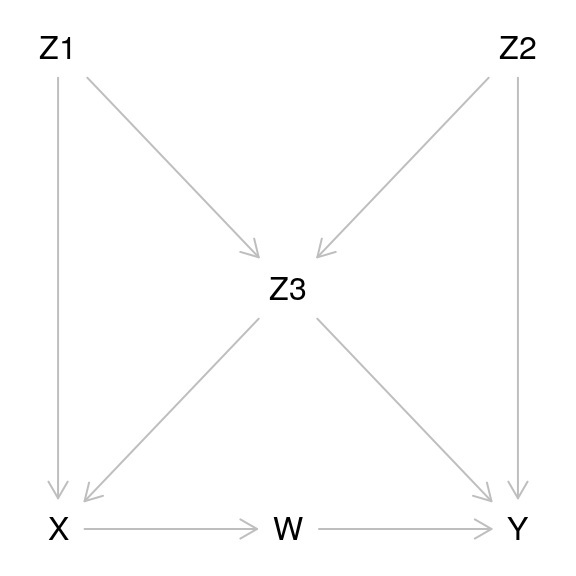Indeed, all edges in the CPDAG remain directed, meaning that no edge can be reversed without generating a new v-structure, destroying an old v-structure, or generating a cycle.

1. Write down a regression equation for 𝑌 such that, if a certain coefficient in that equation is non-zero the model of Figure 2.9 is wrong.

Such regression equations can be derived from conditional independences implied by the model. We can generate a list of such local tests with the following command:

impliedConditionalIndependencies( fig2.9 )
## W _||_ Z1 | X
## W _||_ Z2 | Z1, Z3
## W _||_ Z2 | X
## W _||_ Z3 | X
## X _||_ Y | W, Z2, Z3
## X _||_ Y | W, Z1, Z3
## X _||_ Z2 | Z1, Z3
## Y _||_ Z1 | X, Z2, Z3
## Y _||_ Z1 | W, Z2, Z3
## Z1 _||_ Z2

We can now inspect this list for an independence that involves $$Y$$. For example, we see that $$X$$ and $$Y$$ should be independent given $$W, Z_2, Z_3$$. Hence, when regressing $$Y$$ on $$X,W, Z_2, Z_3$$, the coefficients for $$X$$ must be 0, or else the model is wrong. Let us verify this by simulation. We will generate data from a structural equation model corresponding to Figure 2.9 where all path coefficients are set to 0.4.

d <- simulateSEM( fig2.9, .4, .4 )
coef( summary( lm( Y ~ X + W + Z2 + Z3, d ) ) )
##                 Estimate Std. Error    t value     Pr(>|t|)
## (Intercept)  0.003732794 0.02460160  0.1517297 8.794619e-01
## X           -0.076267761 0.03181000 -2.3976036 1.687224e-02
## W            0.394834468 0.02615344 15.0968437 1.241480e-42
## Z2           0.395466657 0.02658970 14.8729283 1.261246e-41
## Z3           0.448938181 0.03190636 14.0704924 4.580541e-38

Indeed, the X coefficient is not significant, whereas the other ones are.

1. Repeat question (d) for variable $$Z_3$$.

Referring back to the table we generated in (d), we can see that $$Z_3$$ should be independent of $$W$$ given $$X$$. Again, we verify this by simulation:

d <- simulateSEM( fig2.9, .4, .4 )
coef( summary( lm( Z3 ~ X + W, d ) ) )
##                 Estimate Std. Error     t value     Pr(>|t|)
## (Intercept)  0.003820961 0.03876404  0.09856973 9.215196e-01
## X            0.551891598 0.04247616 12.99297212 1.948988e-33
## W           -0.001463496 0.04370156 -0.03348843 9.732985e-01
1. Repeat question (e) assuming the $$X$$ is not measured.

Let us set $$X$$ as an unmeasured variable (latent) and see how that shrinks our list of implied conditional independencies:

latents(fig2.9) <- "X"
impliedConditionalIndependencies( fig2.9 )
## W _||_ Z2 | Z1, Z3
## Y _||_ Z1 | W, Z2, Z3
## Z1 _||_ Z2

There is no longer any implication that renders $$Z_3$$ independent of something else, so we cannot derive such a regression equation anymore.

1. How many regression equations of the type described in (d) and (e) are needed to ensure that the model is fully tested, namely, that if it passes all these tests it cannot be refuted additional tests of these kind.

A set of regression equations like this is called a basis set. We can use the following command to generate, for each variable, the statement that this variable is independent of its non-descendants given its parents. This set constitutes a basis set.

latents(fig2.9) <- c() # undo out setting of X as latent from (f)
impliedConditionalIndependencies( fig2.9, type="basis.set" )
## W _||_ Z1, Z2, Z3 | X
## X _||_ Z2 | Z1, Z3
## Y _||_ X, Z1 | W, Z2, Z3
## Z1 _||_ Z2
## Z2 _||_ Z1

The last two items of the basis set are clearly identical, so we end up with four independence statement. Each of those again corresponds to a regression equation. For instance, the first statement implies that the coefficients of the $$Z$$’s in the following regression must be 0:

d <- simulateSEM( fig2.9, .4, .4 )
coef( summary( lm( W ~ X + Z1 + Z2 + Z3, d ) ) )
##                 Estimate Std. Error     t value     Pr(>|t|)
## (Intercept)  0.081916484 0.04138935  1.97916838 4.835072e-02
## X            0.398483819 0.05810749  6.85770153 2.093583e-11
## Z1          -0.002236596 0.05204057 -0.04297793 9.657365e-01
## Z2           0.019641374 0.04404638  0.44592479 6.558466e-01
## Z3           0.011553227 0.05572433  0.20732824 8.358388e-01

# The Effects of Interventions

## Study question 3.3.1

By now you should have no trouble defining this graph in R. To show yet another option, we will instead download the graph from the dagitty website, where it has been stored for you:

fig3.8 <- downloadGraph("dagitty.net/m331")
plot( fig3.8 )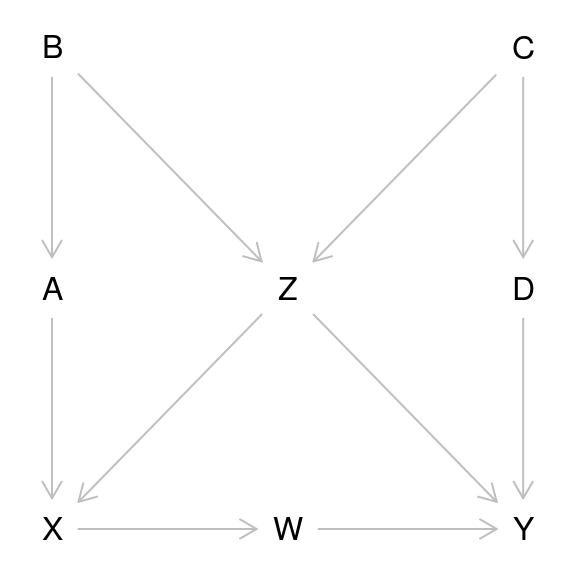1. List all of the sets of variables that satisfy the backdoor criterion to determine the causal effect of $$X$$ on $$Y$$.

This can be done quite easily:

adjustmentSets( fig3.8, "X", "Y", type="all" )
## { A, Z }
## { B, Z }
## { A, B, Z }
## { C, Z }
## { A, C, Z }
## { B, C, Z }
## { A, B, C, Z }
## { D, Z }
## { A, D, Z }
## { B, D, Z }
## { A, B, D, Z }
## { C, D, Z }
## { A, C, D, Z }
## { B, C, D, Z }
## { A, B, C, D, Z }

Note that the back-door criterion is not implemented in dagitty, but instead, a slightly more general criterion that is complete – that is, it is guaranteed to find all set that work to determine the causal effect. Some of these sets can contain descendants of the exposure variable.

1. List all of the minimal sets of variables that satisfy the backdoor criterion to determine the causal effect of $$X$$ on $$Y$$ (i.e., any set of variables such that, if you removed any one of the variables from the set, it would no longer meet the criterion).

This is the default mode of the command adjustmentSets used in the previous answer:

adjustmentSets(fig3.8)
## { D, Z }
## { C, Z }
## { B, Z }
## { A, Z }

This works because the exposure variable $$X$$ and the outcome variable $$Y$$ have already been specified in the graph.

1. List all minimal sets of variables that need be measured in order to identify the effect of $$D$$ on $$Y$$. Repeat, for the effect of $${W, D}$$ on $$Y$$.

To solve these, we now need to re-specify the exposure variable in the graph. This can be done in two ways. Either, we can specify it directly in the graph. Here’s how the first part of this question is solved in that way:

exposures(fig3.8) <- c("D")
adjustmentSets(fig3.8)
## { W, Z }
## { X, Z }
## { A, Z }
## { B, Z }
## { C }

Or we can specify it as an argument to the function adjustmentSets. There is nothing special about specifying two exposures, so we can apply this straightforwardly to solve the second part:

exposures(fig3.8) <- c("W","D")
adjustmentSets(fig3.8)
## { Z }
## { C, X }

## Study question 3.5.1

This study uses the graph of question 3.3.1, which we defined above.

1. Find an expression for the $$c$$-specific effect of $$X$$ on $$Y$$.

We need to find a set that includes $$C$$ and is a valid adjustment set. We can approach this by requiring that $$C$$ be adjusted for:

adjustedNodes(fig3.8) <- c("C")

Now we can list adjustment sets in the same way as before:

adjustmentSets(fig3.8)
## { C, Z }
1. Identify a set of four variables that need to be measured in order to estimate the $$z$$-specific effect of $$X$$ on $$Y$$, and find an expression for the size of that effect.

First we approach this like in (a), and get four different adjustment sets:

adjustedNodes(fig3.8) <- c("Z")
adjustmentSets(fig3.8)
## { D, Z }
## { C, Z }
## { B, Z }
## { A, Z }

Each of these contains only two variables, but we are asked for a set containing four. So let us try combining the first three of those into one. Dagitty features a function that lets us test whether a given set is indeed an adjustment set.

isAdjustmentSet( fig3.8, c("D","C","B","Z") )
##  TRUE

Indeed, this set is a valid adjustment set, and contains four variables as requested. In practice, we may however opt for the smaller set, and especially for the set $${Z,D}$$ which contains a competing exposure for $$Y$$.